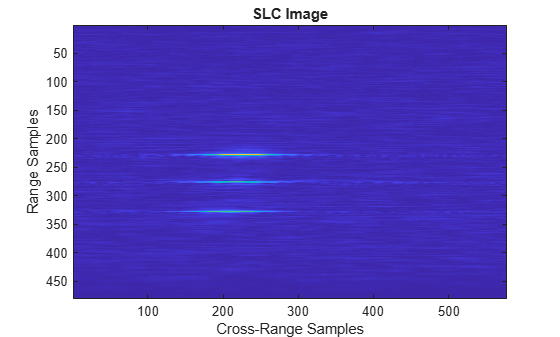# rangeDopplerImagerLFM

Range Doppler image formation algorithm for linear FM waveform

Since R2022a

## Syntax

``slcimg = rangeDopplerImagerLFM(raw,waveform,fc,v,rc)``
``slcimg = rangeDopplerImagerLFM(raw,waveform,fc,v,rc,Name=Value)``

## Description

example

````slcimg = rangeDopplerImagerLFM(raw,waveform,fc,v,rc)` returns a single-look complex image `slcimg` generated from raw synthetic aperture radar (SAR) data obtained using a linear frequency modulated (LFM) pulse `phased.LinearFMWaveform` `waveform`. `fc` specifies the operating frequency. `v` specifies the platform speed. `rc` is the radar-to-beam center distance.```
````slcimg = rangeDopplerImagerLFM(raw,waveform,fc,v,rc,Name=Value)` specifies additional inputs using name-value arguments. Name-value pairs let you specify squint angle, `sqa`, and propagation speed, `c`.```

## Examples

collapse all

Generate a complex-valued single-look SAR image from simulated unfocused raw data. The radar transmits an LFM waveform. The LFM waveform has a 3 microsec duration with a PRF of 960 Hz. The radar has an operating frequency of 9 GHZ with a pulse sweep bandwidth of 29.979 MHz. The radar platform moves at 100 m/s. The radar-to-beamcenter distance is 1.118 km.

FIrst, load the raw image data from a mat-file. The raw data consists of a 481-by-577 complex-valued matrix. Then compute the focused SAR image.

```load('RangeMigrationLFMExampleData.mat') slcimg = rangeDopplerImagerLFM(raw,waveform,fc,v,rc); imagesc(abs(slcimg)) title('SLC Image') xlabel('Cross-Range Samples') ylabel('Range Samples')```## Input Arguments

collapse all

Unfocused IQ raw SAR data, specified as an M-by-N complex-valued matrix. The data is the unfocused in-phase and quadrature (I/Q) raw data collected by the SAR system. Rows of `raw` correspond to along-range samples. The columns of `raw` correspond to the pulses received as the platform moves along the cross-range direction. `raw` must have at least two rows.

Data Types: `double`

Input waveform, specified as a `phased.LinearFMWaveform` object.

Example: `phased.LinearFMWaveform(SampleRate=500e3,'SweepBandwidth',200e3,PulseWidth=1e-3,PRF=1e3)`

Data Types: `double`

Operating frequency, specified as a positive scalar. Units are in Hz.

Example: `2.8e9` Hz specifies a typical S-band operating-frequency value for airport and weather radar systems.

Data Types: `double`

Platform velocity, specified as a positive scalar. Units are in meters per second.

Data Types: `double`

Distance between radar and beam center on the ground, specified as a positive scalar. Units are in meters.

Data Types: `double`

### Name-Value Arguments

Specify optional pairs of arguments as `Name1=Value1,...,NameN=ValueN`, where `Name` is the argument name and `Value` is the corresponding value. Name-value arguments must appear after other arguments, but the order of the pairs does not matter.

Example: `SquintAngle=45,PropagationSpeed=343`

Squint angle of the antenna from the broadside direction in degrees, specified as a scalar in the range `(-90, 90)`.

Data Types: `double`

Signal propagation speed in meters per second, specified as a positive scalar.

Example: `343` meters per second approximates the speed of sound at sea level and at a temperature of 20 °C under normal atmospheric conditions.

Data Types: `double`

## Output Arguments

collapse all

Single-look complex (SLC) image, returned as a matrix. `slcimg` is the same size as `raw` and contains the focused data processed by the range Doppler algorithm.

 Cumming, Ian G., and Frank Hay-chee Wong. Digital Processing of Synthetic Aperture Radar Data: Algorithms and Implementation. Artech House Remote Sensing Library. Boston: Artech House, 2005.

 Curlander, John C., Robert N. McDonough. Synthetic Aperture Radar: Systems and Signal Processing. Wiley, 1991.